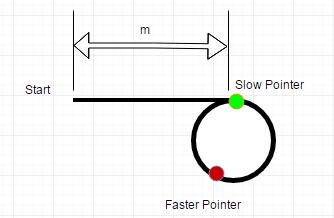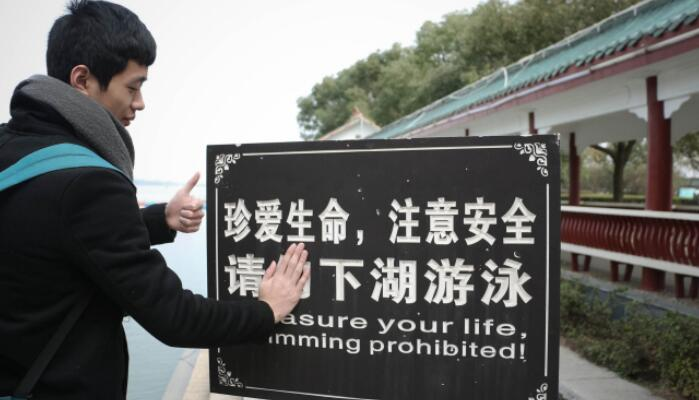In computer science, a linked list is a linear collection of data elements, called nodes, each pointing to the next node by means of a pointer. It is a data structure consisting of a group of nodes which together represent a sequence.A linked list whose nodes contain two fields: an integer value and a link to the next node. The last node is linked to a terminator used to signify the end of the list.2. Classical algorithm problem

Given an linked list, to determine if it has a circle. And try to solve this problem without using extra memory.

There is a technology called fast pointer and slow pointer. The essential key point in this technology is remainder % in mathematic. Each remainder digital number system works like a circle.

Digital System: s = x % y

When y is an integer number which is bigger than 1, any integer number x remaind with y will drop into numbers which’s range is [0, y-1]. Got it ? Yes, It’s like a circle. With the incresement of x, the result of x % y will always drops into [0, y-1]. Let’s back to our initial problem.

So, there will be a circle if two pointer move forward with different speed and they meet each other before the faster pointer get the end of the list. Otherwise, there does not exist a circle.

We can use code to express this algorithm.

How to calculate the beginning location of the circle ?We assume that there exist an circle in a linked list like the picture over there.

Just use two pointer to travel the list.

Hypothesis:

• For the faster pointer, it will move forward two steps each time. For the slower pointer, it will move forward only one steps each time.

• We also assume that m is the distance between the start location of the circle and the start location of the linked list.

• The length of the circle is n.

• The faster pointer will catch the slower pointer after x steps.

According to that hypothesis, we can get some useful information.
When the slower pointer arrive at the start location of the circle, the faster pointer have moved 2m steps. Because they will meet each other after x step for the slower pointer have arrived at the beginning location of the circle. We can make a conclusion.

x / 1 = (n - (m % n) + x)/2

Looking back, they will meet at x = (n - (m % n)) from the beginning location of the circle.

Looking forward, they will meet at x = (m % n) from the beginning location of the circle.

Aha, if we use anther traveller pointer(named jack) move from the original start location after the faster and the slower meet each other. It will walk through m unit of distance.

Make the slower pointer move forward continully. The jack and slow pointer will meet at the beginning point of the circle.

For time costing:

m/1 = m % n

If m < n, the faster will not pass the beginning point more than 1 time after the slower and faster meet, otherwise it will pass the beginning location k times where m = z + k * n(z is the remainder of m % n)

2.2 Find duplicated number

Given an array nums containing n + 1 integers where each integer is between 1 and n (inclusive), prove that at least one duplicate number must exist. Assume that there is only one duplicate number, find the duplicate one.

Note:

You must not modify the array (assume the array is read only). \
You must use only constant, O(1) extra space. \
Your runtime complexity should be less than O(n^2). \
There is only one duplicate number in the array, but it could be repeated more than once. \

We should notice that the range of these integers is [1, n]. And there are n + 1 integers.
We can treat a integer as the index to another integer, which like a pointer.
All integers are store in an array which is a continues memory area.(The implementation of built-in data structure – list is a length-variable array)

That array works like a linked-list, doesn’t it? Every integer can be used as index for the array. And there have one duplicate integer at least, which means that there are at least two “pointer” point to next “node”.

It’s like a single linked-list with circles !

So, the original problem can be transformed into how to find the beginning node of the circle in a linked-list. Just look back 2.1 in this article, if you still don’t know how to do it.

More classical problem wait to be updated :)

LiuYe Lake, HuNan, China.
Photo by Anabella.